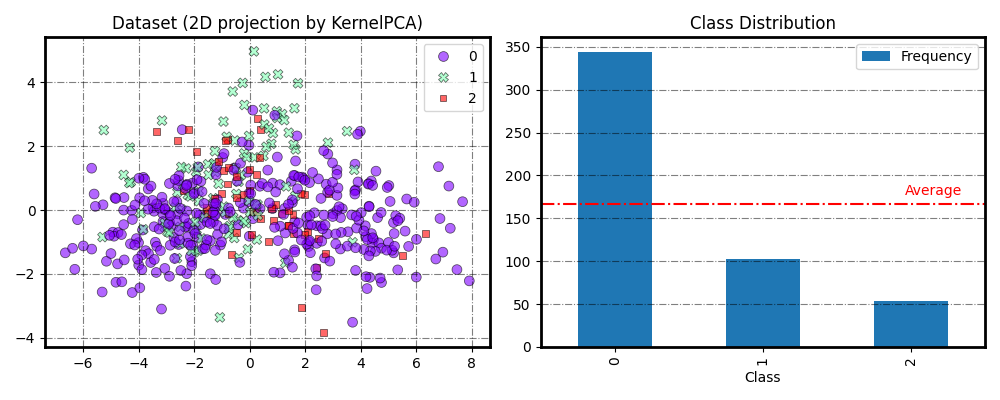# Generate an imbalanced dataset

An illustration of using the `generate_imbalance_data()` function to create an imbalanced dataset.

```# Authors: Zhining Liu <zhining.liu@outlook.com>
```
```print(__doc__)

from imbalanced_ensemble.datasets import generate_imbalance_data
from imbalanced_ensemble.utils._plot import plot_2Dprojection_and_cardinality
from collections import Counter
```

## Generate the dataset

```X_train, X_test, y_train, y_test = generate_imbalance_data(
n_samples=1000, weights=[.7,.2,.1], test_size=.5,
kwargs={'n_informative': 3},
)

print ("Train class distribution: ", Counter(y_train))
print ("Test class distribution:  ", Counter(y_test))
```

Out:

```Train class distribution:  Counter({0: 348, 1: 101, 2: 51})
Test class distribution:   Counter({0: 348, 1: 101, 2: 51})
```

## Plot the generated (training) data

```plot_2Dprojection_and_cardinality(X_train, y_train)
```Out:

```(<Figure size 1000x400 with 2 Axes>, (<AxesSubplot:title={'center':'Dataset (2D projection by KernelPCA)'}>, <AxesSubplot:title={'center':'Class Distribution'}, xlabel='Class'>))
```

Total running time of the script: ( 0 minutes 40.896 seconds)

Estimated memory usage: 21 MB

Gallery generated by Sphinx-Gallery